Courses

# Test: Law Of Mass Action(Chemical Equilibrium)

## 21 Questions MCQ Test Chemistry Class 11 | Test: Law Of Mass Action(Chemical Equilibrium)

Description
This mock test of Test: Law Of Mass Action(Chemical Equilibrium) for JEE helps you for every JEE entrance exam. This contains 21 Multiple Choice Questions for JEE Test: Law Of Mass Action(Chemical Equilibrium) (mcq) to study with solutions a complete question bank. The solved questions answers in this Test: Law Of Mass Action(Chemical Equilibrium) quiz give you a good mix of easy questions and tough questions. JEE students definitely take this Test: Law Of Mass Action(Chemical Equilibrium) exercise for a better result in the exam. You can find other Test: Law Of Mass Action(Chemical Equilibrium) extra questions, long questions & short questions for JEE on EduRev as well by searching above.
QUESTION: 1

### Direction (Q. Nos. 1-12) This section contains 12 multiple choice questions. Each question has four choices (a), (b), (c) and (d), out of which ONLY ONE option is correct.   Q.Conversion factor for converting partial pressures (in Kp) to active masses (in Kc) is

Solution:

Kp = kc(RT)∆ng
So to convert kp to kc we have, kc = kp(1/RT)∆ng.
So, the converting factor is 1/RT

QUESTION: 2

### For which of the following reactions does the equilibrium constant depends on the unit of concentration?

Solution:

For the reaction COCl2 (g) ⇌ CO(g) + Cl2 (g), the equilibrium constant depends on the units of concentration.
For this reaction, the number of moles of reactants is not equal to the number of moles of products. So in the equilibrium constant expression, the units of concentration do not cancel out.
K = [CO] [Cl2] ÷ [COCl2]
K = mol/L × mol/L ÷ mol/L = mol/L

QUESTION: 3

### The concentration of the oxides of nitrogen are monitored in air-pollution reports. At 25°C, the equilibrium constant for the reaction,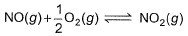is 1.3 x 106 and that for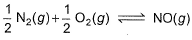is 6.5 x 10-16 (when each species is expressed in terms of partial pressure). For the reaction,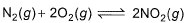equilibrium constant is

Solution:

Given equations are
NO (g) + ½ O2 (g) ⇌ NO2 (g) ----------(i) k1 = 1.3×106
And ½ N2 (g) + ½ O2 (g)     ⇌     NO(g) ----------(ii) k2 = 6.5×10-16
To get the reaction, N2(g) + 2O2(g) ⇌ 2NO2(g) ----------(iii) k3
We multiply eqn (i) and eqn (ii) by 2 and adding both reaction, we get eqn (iii)
k3 = k12×k22
= (1.3×106)2×(6.5×10-16)2
= 1.69×1012×42.25×10-32
= 7.14×10-19

QUESTION: 4

For the following gaseous phase equilibrium,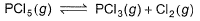Kp is found to be equal to Kx (Kx is equilibrium constant when concentration are taken in terms of mole fraction. This is attained when pressure is

Solution:

The correct answer is Option A.
Kp = Equilibrium constant in terms of partial pressure
Kc = Equilibrium constant in terms of concentration
Kx = Equilibrium constant in terms of mole fraction
Kp = KcRTΔn ---(1)
Kp = K * (Pt)Δn ---(2)
a)   1 atm
Given PCl5 (g) ---> PCl3 (g) + Cl2 (g)
Δn = 2 – 1
Given Kp = Kx
From (2)
Kp = Kx when PT = 1

QUESTION: 5

For the reaction in equilibrium, A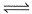B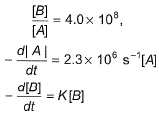Thus, K is

Solution:

From the  reaction
-d[A]/dt = d[B] /dt
⇒2.3 × 106 [A] = k [B]
as given in question [B] /[A] = 4 × 108
so [A] / [B] = 1/ 4 ×108
⇒ 2.3 × 106 . [A] /[B] = k
⇒  2.3 × 106 / 4 × 108 = k
Or k = 5.8 × 10-3 /sec¹

QUESTION: 6

For the following equilibrium, N2O4 (g)2NO2(g)

Kp = KC. This is attained when

Solution:

The correct answer is option B
KP​=KC​(RT)Δn
Δn=1
KP​=KC​(RT)
RT=1
T=1/R=1/0.0821=12.18

QUESTION: 7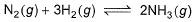is a gaseous phase equilibrium reaction taking place at 400 K in a 5 L flask. For this

Solution:

The correct answer is option B
K, is the equilibrium constant w.r.t the mole fractions of each substance at equilibrium.
Kp = Kc(RT)Δn
Kp = Kx pΔn
by solving it using partial pressure and above formula you get kc=25kx

QUESTION: 8

A sample of pure PCI5(g)was introduced into an evacuated vessel at 473 K. After equilibrium was attained, concentration of PCI5 was found to be 0.05 mol L-1.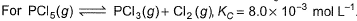Thus, [PCI3] and [Cl2](in mol L-1)at equilibrium are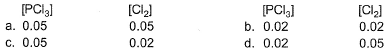Solution:

The correct answer is Option B
Let x M be the equilibrium concentration of PCl3​.
The equilibrium reaction is shown below.
PCl5​(g) ⇌ PCl3​(g) + Cl2​(g)
The equilibrium concentrations of PCl5​, PCl3​ and Cl2​ are 0.5×10−2M, x and x respectively.
The expression of the equilibrium constant is
Kc​= ( [PCl3​][Cl2​] ​) / [PCl3​].
Substitute values in the above expression.
8.3 × 10−3  = x2 / (0.5 × 10−1​)
x2 = 4.15m × 10−4
x ≃ 0.02M.

QUESTION: 9

Ag+(aq)+NH3(aq)[Ag(NH3)(aq)]+ ; K, = 3.5x 10-3
[Ag(NH3)]+ (aq)+NH3(aq)[Ag(NH3)2]2+(aq); K2 = 1.7x 10-3
Formation constant of [Ag(NH3)2]+(aq) is

Solution:

The correct answer is Option C.
To get the formation constant add both reactions-
So the resultant K = K1 × K2
= 3.5×1.7×10−3
= 5.95×10−6

QUESTION: 10

For the reversible reaction,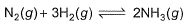at 500° C, the value of Kp is 1.44x 10-5, when partial pressure is measured in atmosphere. The corresponding value of Kc with concentration in mol L-1 is

[IITJEE 2000]

Solution: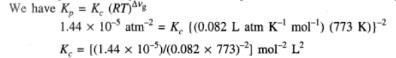QUESTION: 11

For the reaction,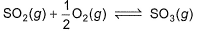if Kp = Kc (RT)X, when the symbols have usual meaning, the value of x is (assuming ideality)

[jee Main 2014]

Solution:

The correct answer is Option B.
SO2(g) + 1/2O2(g) ⇌ SO3(g)
KP = KC(RT)Δn

Δn= no. of gaseous moles of product minus no. of gaseous moles of reactant
Δn = 1−1−1/2
∴Δn = −1/2

QUESTION: 12

Given that for the equilibrium constants of two reactions,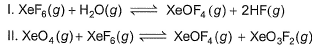areK1 and K2. Equilibrium constant k3 of the following reaction in terms of k1, and K2.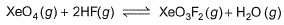Solution:

The correct answer is Option C
XeF6(g) + H2O(g) ⇌ XeOF4(g)+2HF(g)

K1 = ([XeOF4][HF]2 ) / ( [XeF6][H2O] ) ...(i)

XeO4(g) + XeF6(g) ⇌ XeOF4(g) + XeO3F2(g)

K2 = ([XeOF4][XeO3F2] ) / ( [XeO4][XeF6] )  ...(ii)

For the reaction,

XeO4(g) + 2HF(g) ⇌ XeO3F2(g) + H2O(g)

K=[XeO3F2][H2O]) / ([XeO4][HF]2 )  ...(iii)

∴ From Eqs. (i), (ii) and (iii)

K= K2 / K1

*Multiple options can be correct
QUESTION: 13

Direction (Q. Nos. 13-14) This section contains 4 multiple choice questions. Each question has four choices (a), (b), (c) and (d), out of which ONE or  MORE THANT ONE  is correct.

For the following type of reversible reaction,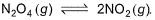Solution:
*Multiple options can be correct
QUESTION: 14

Select the correct statement (s) about the following equilibrium.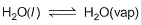Solution:

The correct answers are Options A, B and C.
During constant vapor pressure the no of molecules of H2O leaving the surface = the no of H2O molecules coming to the surface from the atmosphere. This process is dynamic as there is continuous movement of molecules.

QUESTION: 15

Direction (Q. Nos. 15-18) This section contains  a paragraph, wach describing theory, experiments, data etc. three Questions related to paragraph have been given.Each question have only one correct answer among the four given options (a),(b),(c),(d)

Passage I

At 573 K, PCI5 dissociates as,
PCI5 (g) ⇔ PCI3(g)+ Cl2 (g), Kp= 11.5 atm
Given, [PCI3]eq, = [Cl2]eq = 0.01 mol L-1

Q
Kc of this equilibrium is

Solution:

The correct answer is 0.27 mol.L-1
The given reversible gaseous reaction & ice  table:
PCl5(g)⇌PCl3(g) + Cl2(g)
I-  2mol            0 mol       0mol
C- 2α mol        2α mol     2α mol
E- 2(1-α ) mol  2α mol     2α mol
Where degree of dissociation, α=40% =0.4
Volume of the equilibrium mixture, V=2L
At equilibrium the molar concentrations of the components of the mixture are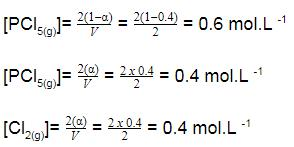Equilibrium Constant: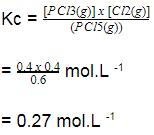QUESTION: 16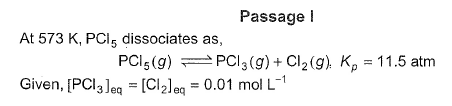Q. What is [PCI5] eq?

Solution:

The correct answer is Option A.
PPCl3 = [PCl3]RT
PCl2 = [Cl2]RT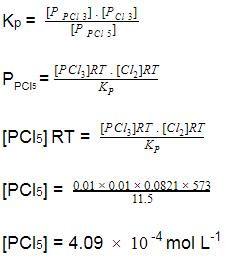QUESTION: 17

Passage II

The complex ion of Fe2+ with the chelating agent dipyridyl (abbreviated dipy) has been studied kinetically in both the forward and backward directions. For the complexion reaction,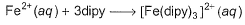the rate of the formation of the complex at 298 K is given by rate = (1. 45 x 1013 L3mol -3s-1) [Fe 2+] [dipy]3 and for the reverse reaction , the rate of disapperance of the complex is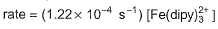Q. What is equilibrium constant for the equilibrium ?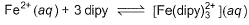Solution:

The correct answer is Option C.
Fe2+ + 3 dipy -----> Fe(dipy)32+
Rf = Kf [Fe2+] [dipy]3
Rb = Kb [Fe(dipy)32+]
Keq = Kb [Fe(dipy)32+] / Kf [Fe2+] [dipy]3
Rf = Rb
Keq = Kf / Kb
= 1. 45 x 1013 / 1.24 x 10-4
= 1.885 x 1017

QUESTION: 18

Passage II

The complex ion of Fe2+ with the chelating agent dipyridyl (abbreviated dipy) has been studied kinetically in both the forward and backward directions. For the complexion reaction,the rate of the formation of the complex at 298 K is given by rate = (1. 45 x 1013 L3mol -3s-1) [Fe 2+] [dipy]3 and for the reverse reaction , the rate of disapperance of the complex isQ. If half-life Period of the backward reaction is(k = rate constant), then half-life period is about

Solution:
*Answer can only contain numeric values
QUESTION: 19

Direction (Q. Nos. 19 and 20) This section contains 2 questions. when worked out will result in an integer from 0 to 9 (both inclusive)

Q. At elevated temperature, PCl5 dissociates as,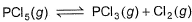At 300°C,Kp = 11.8 and
[PCI3]= [Cl2]= 0.01 moi L-1 at equilibrium.
[PCI5] = x x 10-4 mol L-1 what is the value of x?

Solution:

Applying Kp = kcRT∆ng
11.8 = kc(0.0821)(273+300)(1)
Kc = 0.25
Applying Kc = [PCl3][Cl2]/[PCl5]
0.25 = 10-4 / [PCl5]
[PCl5] = 10-4/0.25
= 4×10-4
Therefore x = 4

*Answer can only contain numeric values
QUESTION: 20

What is Kp for the equation,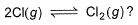When the system contains equal number of Cl (g)atom and Cl2(g) molecules at 1 bar and 300 K?

Solution:

2Cl(g) ⇌ Cl2(g)
Initially take moles of Cl on right hand side to be 1 then
2Cl           Cl2
1               -x
=1 - x.x
kP  will be equal to Kc because no. of moles are equal so
1 - x = x
2x = 1
X = ½
Kp = x/1 - x. 1 - x
= ½ ÷ (½ * ½ )
=2

QUESTION: 21

Direction (Q. Nos. 21) Choice the correct combination of elements and column I and coloumn II  are given as option (a), (b), (c) and (d), out of which ONE option is correct.

The progress of the reaction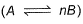with time t is shown below.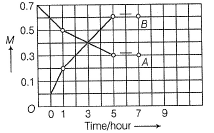Match the parameters in Column l with their respective values in Column II.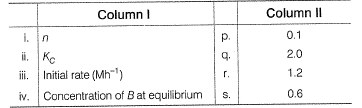Codes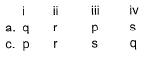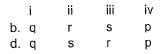Solution:

The correct answer is Option A.

Loss in concentration of A in I hour =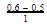= 0.1
Gain in concentration of B in I hour =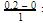0.2
(i) ∵0.1 mole of A changes to 0.2 mole of B in a given time and thus, n=2
(ii) Equilibrium constant,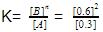= 1.2mollitre−1
(iii) Initial rate of conversion of A = changes in conc. of A during I hour =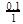= 0.1 mol litre−1hour−1
(iv) ∵ Equilibrium is attained after 5 hr, where [B]=0.6 and [A]=0.3